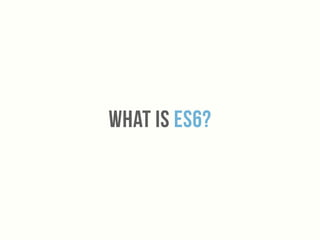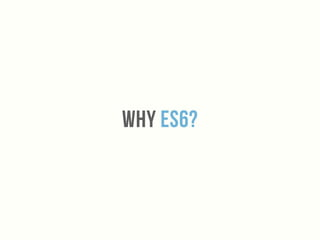Diese Präsentation wurde erfolgreich gemeldet.

# Impress Your Friends with EcmaScript 2015

Anzeige
Anzeige
Anzeige
Anzeige
Anzeige
Anzeige
Anzeige
Anzeige
Anzeige
Anzeige
Anzeige×

1 von 79 Anzeige

# Impress Your Friends with EcmaScript 2015

Here are the slides that I gave for The Arizona Software Community meetup.

http://www.meetup.com/azsoftcom/events/222936544/

This was a gentle introduction to some of the features in EcmaScript 2015 and how and why you may use them.

Here are the slides that I gave for The Arizona Software Community meetup.

http://www.meetup.com/azsoftcom/events/222936544/

This was a gentle introduction to some of the features in EcmaScript 2015 and how and why you may use them.

Anzeige
Anzeige

### Impress Your Friends with EcmaScript 2015

1. 1. EcmaScript 2015impress your friends at the party
2. 2. What is es6?
3. 3. Why es6?
4. 4. Is it Safe to Us Es6?!?!
5. 5. https://kangax.github.io/compat-table/es6/
6. 6. How do we use Es6?
7. 7. https://github.com/rauschma/webpack-es6-demo
8. 8. https://github.com/angular-class/NG6-starter
9. 9. Syntactic SugAr
10. 10. Es6 Classes
11. 11. ES6 Classes • Simple sugar over the the prototype-based OO pattern • A more convenient form makes classes easier to use • Classes support inheritance, super calls, instance and static methods and constructors
12. 12. class Project {  constructor(name) {  this.name = name;  }    start() {  return `Project \${this.name} starting`;  }  }    var project = new Project("Website");  project.start(); // "Project Website starting"
13. 13. Es6 Inheritance
14. 14. ES6 INheritance • Inheritance is possible via the prototype • You can inherit from a base class using the extends keyword • You must call super before you can access this in a subclass
15. 15. class Shape {  …  toString () {  return `Shape(\${this.id})`  }  }  class Rectangle extends Shape {  constructor (id, x, y, width, height) {  super(id, x, y)  …  }  toString () {  return "Rectangle > " + super.toString()  }  }  class Circle extends Shape {  constructor (id, x, y, radius) {  super(id, x, y)  …  }  toString () {  return "Circle > " + super.toString()  }  }
16. 16. Es6 Modules
17. 17. ES6 MODULES • Language-level support for modules for component deﬁnition • Best of both both CommonJS and AMD • Named exports and default exports
18. 18. //------ lib.js ------  export const sqrt = Math.sqrt;  export function square(x) {  return x * x;  }  export function diag(x, y) {  return sqrt(square(x) + square(y));  }    //------ main.js ------  import { square, diag } from 'lib';  console.log(square(11)); // 121  console.log(diag(4, 3)); // 5
19. 19. //------ main.js ------  import * as lib from 'lib';  console.log(lib.square(11)); // 121  console.log(lib.diag(4, 3)); // 5
20. 20. //------ myFunc.js ------  export default function () { ... };    //------ main1.js ------  import myFunc from 'myFunc';  myFunc();
21. 21. //------ MyClass.js ------  export default class { ... };    //------ main2.js ------  import MyClass from 'MyClass';  let inst = new MyClass();
22. 22. Es6 Block Level Scoping
23. 23. ES6 MODULES • Function scope can be tricky! • We can scope variables at a block level using let and const
24. 24. function printName() {  if(true) {  var name = "Rafael";  }  console.log(name); // Rafael  }
25. 25. function printName() {  var name; // variable declaration is hoisted to the top  if(true) {  name = "Rafael";  }  console.log(name); // Rafael  }
26. 26. function printName() {  if(true) {  let name = "Rafael";  }  console.log(name); // ReferenceError: name is not defined  }
27. 27. function printName() {  var name = "Hey";  if(true) {  let name = "Rafael";  console.log(name); // Rafael  }  console.log(name); // Hey  }
28. 28. if (true) { // enter new scope, TDZ starts    // Uninitialized binding for `tmp` is created  tmp = 'abc'; // ReferenceError  console.log(tmp); // ReferenceError    let tmp; // TDZ ends, `tmp` is initialized with `undefined`  console.log(tmp); // undefined    tmp = 123;  console.log(tmp); // 123  }
29. 29. Es6 Arrow functions
30. 30. ES6 Arrow Functions • Arrow function expression aka fat arrow functions are a shorter syntax • Lexically binds the this value • Arrow functions are always anonymous
31. 31. var numbers = [1,2,3,4,5];  var timesTwo = numbers.map(function (number) {  return number * 2;  });  console.log(timesTwo); // [2, 4, 6, 8, 10]
32. 32. var numbers = [1,2,3,4,5];  var timesTwo = numbers.map((number) => number * 2);  console.log(timesTwo); // [2, 4, 6, 8, 10]
33. 33. var numbers = [1,2,3,4,5];  var timesTwo = numbers.map(number => number * 2);  console.log(timesTwo); // [2, 4, 6, 8, 10]
34. 34. function FooCtrl (FooService) {  this.foo = 'Hello';  FooService  .doSomething(function (response) {  this.foo = response;  });  }
35. 35. function FooCtrl (FooService) {  this.foo = 'Hello';  FooService  .doSomething(function (response) {  this.foo = response;  }.bind(this));  }
36. 36. function FooCtrl (FooService) {  var that = this;  that.foo = 'Hello';  FooService  .doSomething(function (response) {  that.foo = response;  });  }
37. 37. function FooCtrl (FooService) {  this.foo = 'Hello';  FooService  .doSomething((response) => { // woo, pretty  this.foo = response;  });  }
38. 38. function FooCtrl (FooService) {  this.foo = 'Hello';  FooService  .doSomething(response => this.foo = response);  }
39. 39. Es6 Template Strings
40. 40. ES6 Template strings • Multi-line strings • Expression interpolation • Do not let untrusted users construct template strings!
41. 41. console.log("string text line 1n"+  "string text line 2");  // "string text line 1  // string text line 2"
42. 42. console.log(`string text line 1  string text line 2`);  // "string text line 1  // string text line 2"
43. 43. var a = 5;  var b = 10;  console.log("Fifteen is " + (a + b) + " andnnot " + (2 * a + b) + ".");  // "Fifteen is 15 and  // not 20."
44. 44. var a = 5;  var b = 10;  console.log(`Fifteen is \${a + b} andnnot \${2 * a + b}.`);  // "Fifteen is 15 and  // not 20."
45. 45. Es6 Object Literals
46. 46. ES6 Object Literals • Object literals are extended to be more convenient and more like deﬁning a class • Better property and method declarations
47. 47. var x = 10;  var y = 30;    var coordinates = {  x: x,  y: y  };    console.log(coordinates); // { x: 10, y: 30 }
48. 48. let x = 10;  let y = 30;    let coordinates = { x, y };    console.log(coordinates); // { x: 10, y: 30 }
49. 49. let x = 10;  let y = 30;    let coordinates = {  x,  y,  z: 10,  };    console.log(coordinates); // { x: 10, y: 30, z: 10 }
50. 50. var cart = {  _items: [],  addItem: function(item) {  this._items.push(item);    return this;  },  toString: function() {  return this._items.join(', ');  }  }    cart.addItem('apple')  .addItem('orange')  .addItem('banana');    console.log(cart.toString()); // apple, orange, banana
51. 51. var cart = {  _items: [],  addItem(item) {  this._items.push(item);    return this;  },  toString() {  return this._items.join(', ');  }  }    cart.addItem('apple')  .addItem('orange')  .addItem('banana');    console.log(cart.toString()); // apple, orange, banana
52. 52. Es6 Array API
53. 53. ES6 Array API • Array.from converts array-like objects into arrays • Array.keys, Array.values and Array.entries are handy for iterating over arrays • Array.ﬁnd returns the ﬁrst element that the callback returns true •Array.ﬁndIndex returns the index of the ﬁrst element that the callback returns true
54. 54. // Array-like object (arguments) to Array  function f() {  return Array.from(arguments);  }    f(1, 2, 3);  // [1, 2, 3]      // Any iterable object...  // Set  var s = new Set(["foo", window]);  Array.from(s);  // ["foo", window]      // Map  var m = new Map([[1, 2], [2, 4], [4, 8]]);  Array.from(m);  // [[1, 2], [2, 4], [4, 8]]      // String  Array.from("foo");  // ["f", "o", "o"]
55. 55. var arr = ["a", "b", "c"];  var iterator = arr.keys();    console.log(iterator.next()); // { value: 0, done: false }  console.log(iterator.next()); // { value: 1, done: false }  console.log(iterator.next()); // { value: 2, done: false }  console.log(iterator.next()); // { value: undefined, done: true }
56. 56. var arr = ['w', 'y', 'k', 'o', 'p'];  var eArr = arr.values();  console.log(eArr.next().value); // w  console.log(eArr.next().value); // y  console.log(eArr.next().value); // k  console.log(eArr.next().value); // o  console.log(eArr.next().value); // p
57. 57. var arr = ['a', 'b', 'c'];  var eArr = arr.entries();    console.log(eArr.next().value); // [0, 'a']  console.log(eArr.next().value); // [1, 'b']  console.log(eArr.next().value); // [2, 'c']
58. 58. function isPrime(element, index, array) {  var start = 2;  while (start <= Math.sqrt(element)) {  if (element % start++ < 1) {  return false;  }  }  return element > 1;  }    console.log([4, 6, 8, 12].find(isPrime)); // undefined, not found  console.log([4, 5, 8, 12].find(isPrime)); // 5
59. 59. function isPrime(element, index, array) {  var start = 2;  while (start <= Math.sqrt(element)) {  if (element % start++ < 1) {  return false;  }  }  return element > 1;  }    console.log([4, 6, 8, 12].findIndex(isPrime)); // -1, not found  console.log([4, 6, 7, 12].findIndex(isPrime)); // 2
60. 60. Es6 SET
61. 61. ES6 SET • Lets you store unique values of any type • You can store primitive values or object references
62. 62. var mySet = new Set();    mySet.add(1);  mySet.add(5);  mySet.add("some text");    mySet.has(1); // true  mySet.has(3); // false, 3 has not been added to the set  mySet.has(5); // true  mySet.has(Math.sqrt(25)); // true  mySet.has("Some Text".toLowerCase()); // true    mySet.size; // 3    mySet.delete(5); // removes 5 from the set  mySet.has(5); // false, 5 has been removed    mySet.size; // 2, we just removed one value
63. 63. Es6 MAP
64. 64. ES6 MAP • Simple key/value map • Any value (object or primitive value) can be used as either a key or a value
65. 65. var myMap = new Map();    var keyObj = {},  keyFunc = function () {},  keyString = "a string";    // setting the values  myMap.set(keyString, "value associated with 'a string'");  myMap.set(keyObj, "value associated with keyObj");  myMap.set(keyFunc, "value associated with keyFunc");    myMap.size; // 3    // getting the values  myMap.get(keyString); // "value associated with 'a string'"  myMap.get(keyObj); // "value associated with keyObj"  myMap.get(keyFunc); // "value associated with keyFunc"    myMap.get("a string"); // "value associated with 'a string'"  // because keyString === 'a string'  myMap.get({}); // undefined, because keyObj !== {}  myMap.get(function() {}) // undefined, because keyFunc !== function () {}
66. 66. Es6 Resources
67. 67. https://leanpub.com/exploring-es6/
68. 68. http://es6-features.org/
69. 69. http://es6katas.org/
70. 70. http://www.es6fiddle.net/ http://es6-features.org/ http://www.2ality.com/ https://github.com/lukehoban/es6features http://codepen.io/bradleyboy/posts/getting-to-know-es6-object-literals http://jamesknelson.com/es6-the-bits-youll-actually-use http://jamesknelson.com/es6-cheatsheet.png
71. 71. Thank you!